Mobile QR Code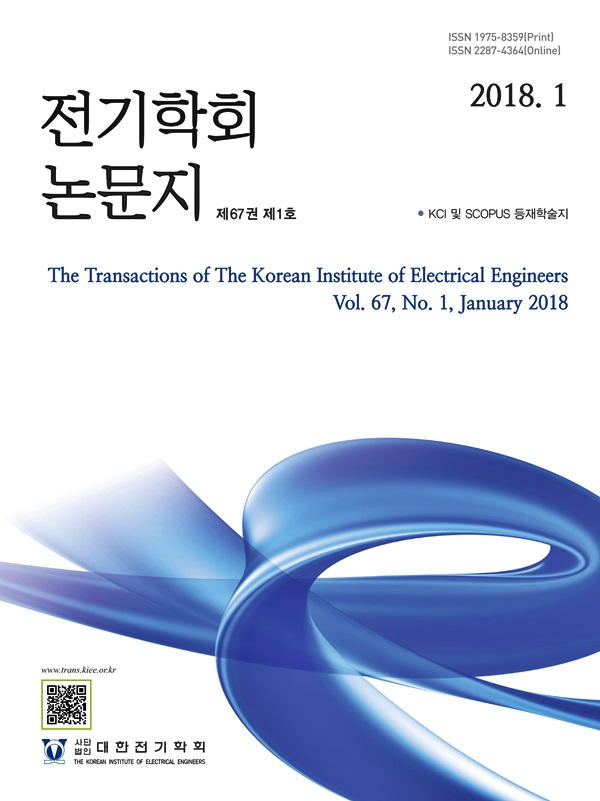#### The Transactions of the Korean Institute of Electrical Engineers

##### ISO Journal TitleTrans. Korean. Inst. Elect. Eng.

1. (Dept. of Electrical& Electronics and Communication Engineering, Koreatech University, Korea. Dep. of Electrical Engineering, Assiut University, Assiut, Egypt.)

AC power supply, Inductive load, Small signal models, PFC, VSI with LC filter, Power factor, THD

## 1. Introduction

The reactive power required for magnetization increases the amounts of required supply apparent power to the distribution system in industrial inductive loads such as electric motors, inductors, transformers, and contactor coils. The input PF will be reduced as the supply reactive and apparent power are increased. Reduced PF causes larger conductors, large copper losses, poor voltage regulation, and a reduction in system power and current capacity (1).

Many techniques have recently been used to improve system power performance and stability with these loads, such as reactive power compensation techniques using flexible AC transmission systems (FACTS) devices such as static var compensator (SVC), static synchronous series compensator (SSSC), and static synchronous compensator (STATCOM), which are used to improve system operation and stability by

Fig. 1. Structure of the proposed two-stage AC-AC converter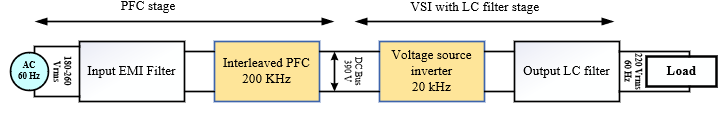controlling the active-reactive power flow on the power grid with such these loads. Active power filters also widely used current injection and reactive power compensation to aid in harmonic current compensation (6), (7).

As shown in Fig 1, a two-stage AC-AC power supply based on rectifying AC power into DC and then DC into AC with an inverter circuit can be used to control the input PF, reduce THD values at the supply and load sides, and regulate the voltage and frequency to distribution levels which capable of handling the operation of the power system with such loads.

The power factor correction stage (PFC) is the first stage of the power supply and usually implements two control loops, one for the current to keep the phase shift between the supply voltage and current waveforms at the lowest value and thus keep the input power factor at the highest value, and another for the voltage to keep the output voltage at the required DC bus voltage. Many PFC converter circuit typologies are presented in (8), (9).

Most of these typologies are based on the boost converter operation principle, such as conventional boost PFC, interleaved boost PFC, and bridgeless boost PFC converters. When compared to conventional and bridgeless PFC boost converters, the interleaved PFC boost converter provides conversion with input ripple current cancellation, which reduces current THD, current stress in the converter components, power losses, and increases conversion efficiency. Furthermore, interleaving the input current into two channels and collecting it again on the output side reduces thermal stress in the converter components, improving thermal performance and increasing conversion capacity (9)-(12).

The proposed power supply's second stage is implemented as a voltage source inverter (VSI), which has recently been used to improve power quality in many industrial applications (13)-(15). A VSI with optimally designed two control loops and an LC filter can be used to convert the front stage's DC bus voltage to the required AC distribution level on the load side, as well as to maintain harmonics contents and ripple current values at the specified levels.

The rest of this paper is organized as follows: Section 2 describes the design of the power components used to derive the small-signal model based on the averaging small signal stability technique, as well as the voltage and current control loops of the interleaved PFC stage. Section 3 presents the small-signal model of the VSI stage, control loops designed using the Ziegler Nichols closed-loop tuning technique, and the optimal design of the LC filter components. Section 4 displays the modeling and simulation results for the proposed two-stage power supply. Section 5 is the conclusion of the paper.

## 2. Interleaved PFC Stage

Fig 2 depicts the schematic circuit of the employed power supply's front stage, which is a 2-channel interleaved PFC boost converter stage comprised of an EMI filter, a bridge rectifier, and a symmetric 2-unit of a single-phase boost converter operating with a 180-degree phase shift.

The interleaved PFC boost converter's operation principle is based on the operation of the 2-channel interleaved boost converter shown in Fig 3. The interleaved boost converters, inductors, and bulk capacitor must be optimally designed in order to keep the voltage and current ripples within the limits specified in the following subsections.

Fig. 3. Schematic circuit of the interleaved boost converter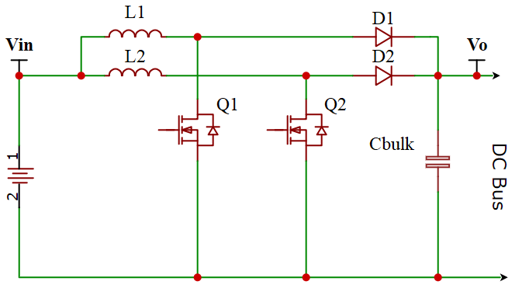### 2.1 Interleaved Boost inductors and bulk capacitor design

Given that the 2-unit is symmetric and that the PWM of each unit has a 180-degree phase shift. The circuit operation of the designed interleaved boost converter working in continuous current conduction mode (CCM) can represent four operating modes, and if the average duty cycle is less than 0.5, both switches will not be turned on at the same time, resulting in three operating modes that describe the on-off states of the 2-channel switches as depicted in the converter waveforms in Fig 4, where $V_{L1}$, $V_{L2}$ represent inductors voltages and $I_{L1}$, $I_{L2}$ represent the inductors currents.

The employed interleaved boost converter is designed to work with a wide range of input AC supply voltages ranging from 180V to approximately 260V rms, and the power components of the boost converter unit are designed to offer good performance with the lowest input voltage level where the operating duty cycle can be calculated as:

##### (1)
$D_{on}=\dfrac{V_{o}-Vin\min\times √2}{V_{o}}$

The boost inductor ripple current peak to peak value can be calculated as the percentage (% Ripple) of the converter total input current.

##### (2)
$\triangle I_{Lrpk}=\dfrac{P_{out}\times √2\times\%Ripple}{Vin\min\times\eta\times K}$

The variable K is defined as the normalized input ripple current factor for interleaved boost converters and can be calculated as follow:

##### (3)
K=\sum\begin{aligned}\dfrac{1-2D}{1-D} {if}D\le 0.5\\\dfrac{2D-1}{D} {if}D\ge 0.5\end{aligned}

For the interleaved boost the boost inductor at each channel can calculated as:

##### (4)
$L_{1}=L_{2}=\dfrac{Vin\min\times √2\times D_{on}}{\triangle I_{Lrpk}\times F_{sw}}$

The output capacitor value usually calculated to meet the specified output voltage ripple using (5), also, this value should be enough to deliver the output minimum voltage hold up with the specific time ($t_{hold}$), as given in (6) where $V_{rpp}$ is the peak to peak output voltage ripple. The larger value among the two equations was selected to design the output capacitor.

##### (5)
$C_{bulk}\ge \dfrac{P_{o}}{2\times\pi\times F\times V_{rpp}\times V_{o}}$

##### (6)
$C_{bulk}\ge \dfrac{2\times P_{o}\times t_{hold}}{V_{o}^{2}-V_{o\min}^{2}}$

Using the converter parameters given in Table 1, the required boost inductors, and bulk capacitance for the interleaved boost converter can be obtained.

Table 1. Design specifications of the PFC stage

 Design Specifications Parameter Value Unit Supply voltage ($V_{in}$) 220 (180-260) V Supply Frequency (F) 60 Hz Output Voltage ($V_{o}$) 390 (320-410) V Rated Power (Po) 300 W Desired Efficiency (𝜼) 95% - Switching Frequency ($F_{sw}$) 200 kHz Hold Up Time ($t_{hold}$) 10 ms Inductor ripple (% Ripple) 30% voltage ripple ($V_{rpp}$) 16 Vpp

### 2.2 Interleaved boost converter small signal modeling

Assume the interleaved boost converter used in this work is in continuous current conduction (CCM) mode. The mathematical equations that describe the change in inductors currents ($I_{L1}$, $I_{L2}$) and output voltage ($V_{o}$) in the converter depicted in Fig 3 are as follow:

##### (7)
$\dfrac{d I_{L1}(t)}{dt}=\dfrac{Vin}{L_{1}}-\dfrac{V_{o}(1-D)}{L_{1}}$

##### (8)
$\dfrac{d I_{L2}(t)}{dt}=\dfrac{Vin}{L_{2}}-\dfrac{V_{o}(1-D)}{L_{2}}$

##### (9)
$\dfrac{d V_{o}(t)}{dt}=\dfrac{I_{L1}(1-D)}{C_{bulk}}+\dfrac{I_{L2}(1-D)}{C_{bulk}}-\dfrac{V_{o}}{RC_{bulk}}$

Assume all variables $\left(I_{L1},\:I_{L2},\:Vin ,\:V_{o},\:{and}D\right)$are at the steady state at the selected operational point and the small-signal AC variation $\left .(i_{L1}^{*},\:i_{L2}^{*},\:v_{i n}^{*},\:v_{o}^{*},\:{and}d^{*}\right)$, where

##### (10)
\begin{align*} I_{L1}=i_{L1}+i_{L1}^{*};I_{L2}=i_{L2}+i_{L2}^{*};\\ Vin =Vin +Vin *;V_{o}=v_{o}+v_{o}^{*};D=d+d^{*} \end{align*}

Substituting from (10) in (7)– (9), and with using the averaging small signal technique (9), which assume that over the switching period, the change in the inductor current and the change in capacitor voltage is equal to zero we obtain

##### (11)
$\dfrac{d\left(i_{L1}^{*}\right)}{dt}=\dfrac{1}{L_{1}}(Vin *)+\dfrac{v_{o}}{L_{1}}\left(d^{*}\right)-\dfrac{(1-d)}{L_{1}}\left(v_{o}^{*}\right)$

##### (12)
$\dfrac{d\left(i_{L2}^{*}\right)}{dt}=\dfrac{1}{L_{2}}(Vin *)+\dfrac{v_{o}}{L_{2}}\left(d^{*}\right)-\dfrac{(1-d)}{L_{2}}\left(v_{o}^{*}\right)$

##### (13)
\begin{align*} \dfrac{d\left(v_{o}^{*}\right)}{dt}=\dfrac{(1-d)}{C_{bulk}}\left(i_{L1}^{*}\right)+\dfrac{(1-d)}{C_{bulk}}\left(i_{L2}^{*}\right)\\ +\dfrac{i_{L1}+i_{L1}}{C_{bulk}}\left(d^{*}\right)-\dfrac{1}{RC_{bulk}}\left(v_{o}^{*}\right) \end{align*}

Arrange state Equations (11)–(13) in state space matrix form to obtain the state space representation of the interleaved CCM PFC converter's small signal stability model as (14).

##### (14)
$\left[\begin{array}{c}\dot{i}_{L 1}^{*} \\ \dot{i}_{L 2}^{*} \\ v_{0}^{*}\end{array}\right]=\left[\begin{array}{ccc}0 & 0 & -\frac{(1-d)}{L_{1}} \\ 0 & 0 & -\frac{(1-d)}{L_{2}} \\ \frac{(1-d)}{C_{b u l k}} & \frac{(1-d)}{C_{b u l k}}-\frac{1}{R C_{b u l k}}\end{array}\right]\left[\begin{array}{c}i_{L 1}^{*} \\ i_{L 2}^{*} \\ v_{o}^{*}\end{array}\right]$ $+\left[\begin{array}{cc}\frac{1}{L_{1}} & \frac{v_{o}}{L_{1}} \\ \frac{1}{L_{2}} & \frac{v_{o}}{L_{2}} \\ 0 & \frac{i_{L 1}+i_{L 2}}{C_{b}}\end{array}\right]\left[\begin{array}{c}v i n^{*} \\ d^{*}\end{array}\right]$

### 2.3 Control Circuits Design of the PFC Stage

Fig. 5. The structure of the control system of the PFC stage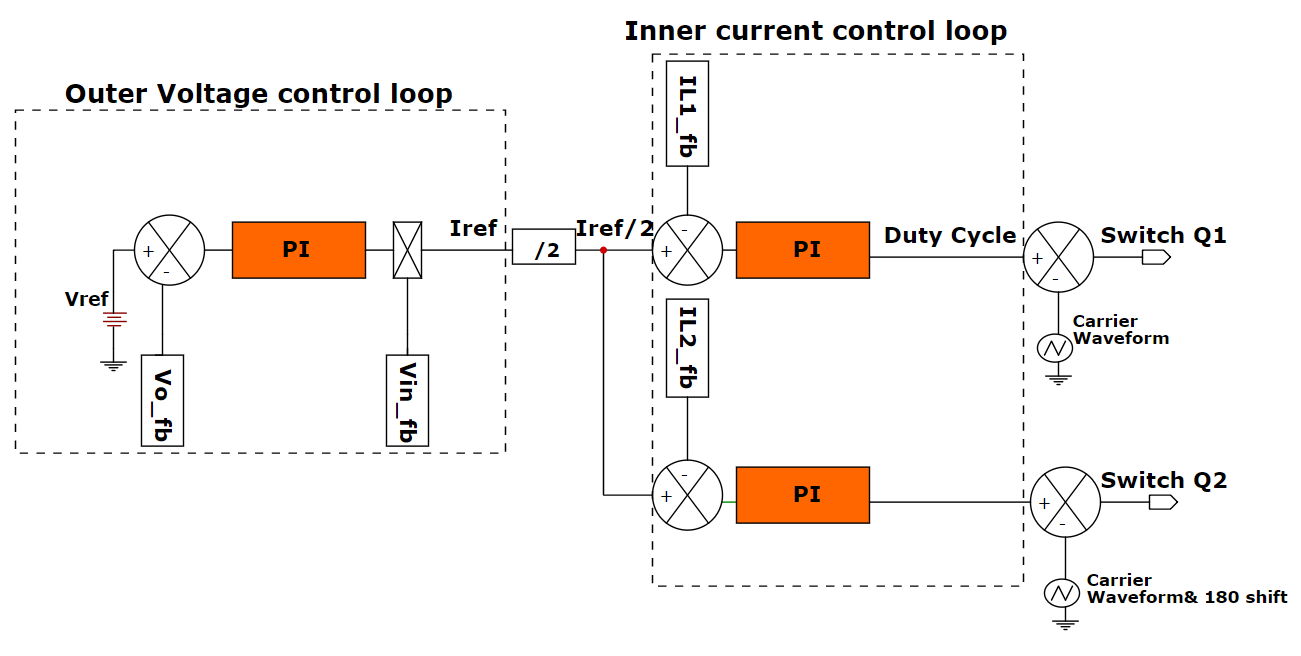Using the Laplace transform of the small signal model obtained in (14), the transfer functions (TF) of the output voltage and inner current control systems for the interleaved CCM PFC converter can be obtained as (15), (16). The TF of the PI controllers for the voltage and current control loops can also be expressed as (17). (18).

##### (15)
$G_{v}(s)=\dfrac{v_{o}^{*}(s)}{i_{L1,\:2}^{*}(s)}=\dfrac{Vin}{2V_{o}C_{bulk}s}$

##### (16)
$G_{i}(s)=\dfrac{i_{L1,\:2}^{*}(s)}{d^{*}(s)}=\dfrac{\left(\dfrac{V_{o}}{L_{1,\:2}C_{bulk}R}\right).\left(s RC_{bulk}+2\right)}{s^{2}+\dfrac{1}{C_{bulk}R}s+\dfrac{2}{L_{1,\:2}C_{bulk}}(1-d)^{2}}$

##### (17)
$G_{P Iv}(s)=K_{Pv}+\dfrac{K_{Iv}}{s}$

##### (18)
$G_{P Ii}(s)=K\pi +\dfrac{K_{Ii}}{s}$

Because the converter switching frequency ($F_{sw}$) in this work was chosen to be around 200kHz for the inner current control loops, the capacitor ($C_{bulk}$) can be shorted for high frequency analysis, and the open-loop TF of the inductor current system in (19) can be simplified as follows (19).

##### (19)
$G_{i1}(s)=G_{i2}(s)=\dfrac{2V_{o}}{sL_{1,\:2}}$

Fig. 6. Closed loop voltage control system of the PFC stage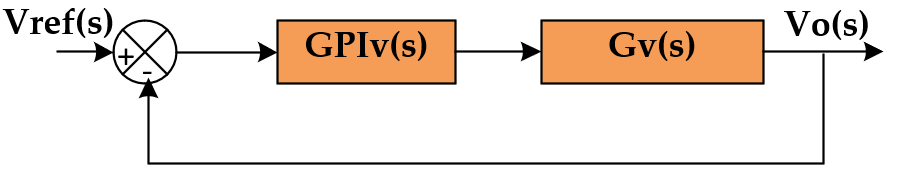Fig 6 depicts the control blocks of the interleaved PFC stage's closed loop voltage control system. Where the voltage closed loop's TF can be expressed as:

##### (20)
$G_{clv}=\dfrac{\dfrac{v_{i n}K_{Pv}}{2V_{o}C_{bulk}s}s+\dfrac{v_{i n}K_{Iv}}{2V_{o}C_{bulk}s}}{s^{2}+\dfrac{v_{i n}K_{Pv}}{2V_{o}C_{bulk}s}+\dfrac{vi nK_{Iv}}{2V_{o}C_{bulk}s}}$

Fig. 7. Closed loop current control system of the PFC stage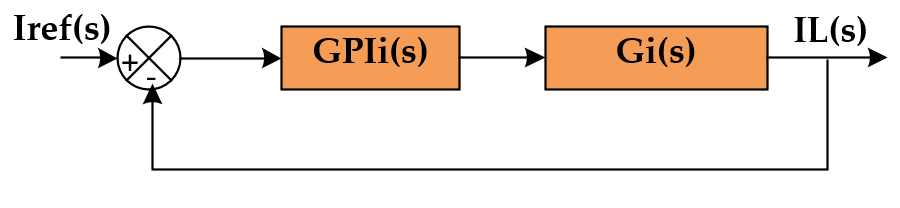Fig 7 depicts the control blocks of the interleaved PFC stage's closed loop current control system. Where the current closed loop's TF can be expressed as:

##### (21)
$G_{cli}(s)=\dfrac{\dfrac{2V_{o}K_{P i}}{L_{1,\:2}}s+\dfrac{2V_{o}K_{Ii}}{L_{1,\:2}}}{s^{2}+\dfrac{2V_{o}K_{P i}}{L_{1,\:2}}s+\dfrac{2V_{o}K_{Ii}}{L_{1,\:2}}}$

Once the transfer functions of the control loops have been determined, the gains of the PI controller can be obtained using the standard form of the second-order system TF by selecting the appropriate control system bandwidth and undamped natural frequency, which improved the optimal stability criterion. To avoid harmonics of the output DC voltage reflected by the AC input voltage at 60Hz, the outer voltage loop's bandwidth must typically be very small (9). Also, the bandwidth of the inner current loop must be greater than that of the outer voltage control loop in order for the inductor current to follow the reference current. In this study, the undamped natural frequency (ξ) was assumed to be around 0.707, and the closed-loop bandwidth values for the interleaved PFC converter's outer voltage and inner current loops were assumed to be around 100rad/s and 50,000rad/s, respectively.

Fig 8 and Fig 9 show the bode plots of the closed-loop TF of the control loops, which show that the closed loop voltage controller provides unity gain for frequencies less than 35Hz. This voltage controller functions as a low pass filter to remove the 60Hz voltage ripple. In addition, for frequencies less than 16.3kHz, the current control controller acts as a low pass filter, rejecting current ripples reflected at the switching frequency.

Fig. 8. Bode plot of the PFC stage voltage control system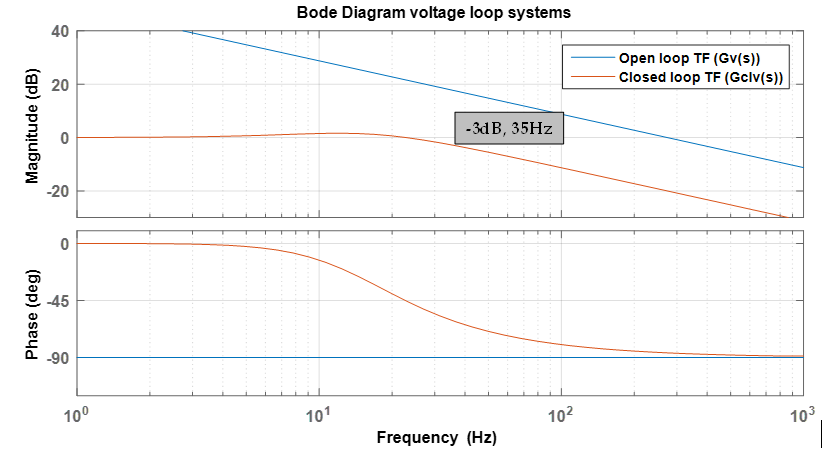Fig. 9. Bode plot of the PFC stage current control system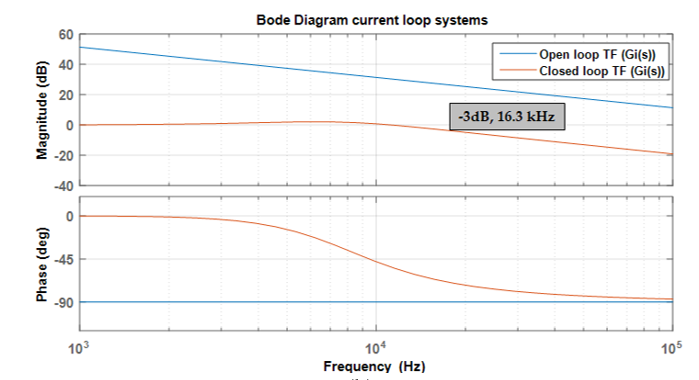## 3. VSI with LC Filter Stage

The schematic circuit of a full-bridge voltage source inverter (VSI) with an output LC filter is shown in Fig 10 , and the design of the LC filter components and the control circuit of the full-bridge switches are presented in the following subsections.

Switches Q3 and Q4 operate at high frequencies, while switches Q5 and Q6 operate at low frequencies, resulting in uni-polar modulation. Table 2 lists the switching states used on this reference design. Ascertain that the PWM waveform is symmetric around the zero crossing of the AC wave.

Table 2. VSI stage full bridge PWM sequence

 Mode Cycle Q3 Q4 Q5 Q6 Vinv 1 +Ve half cycle on off off on Vbus 2 +Ve half cycle off on off on 0 3 -Ve half cycle off on on off -Vbus 4 -Ve half cycle on off on off 0

### 3.1 Design of the Output LC Filter

The filter inductance is intended to control the current ripple on the VSI's output load side. To determine the optimal value of the filter inductance, the modulation index at the maximum ripple current is calculated. The inductor ripple current is expressed as:

##### (22)
$V_{bus}-V_{Load}=L_{f}\times\dfrac{\triangle i_{Lfrpp}}{D\times T_{s}}$

##### (23)
$\triangle i_{Lfrpp}=\dfrac{D\times T_{s}\times\left(V_{bus}-V_{Load}\right)}{L_{f}}$

The duty cycle is calculated using the modulation index (mi) of the VSI as:

##### (24)
$D(\omega t)=m_{i}\times\sin\omega t$

It is safe to presume that the inverter's output will match the AC voltage:

##### (25)
$V_{Load}=V_{bus}\times D$

With substituting from (26), (27) in (25), the inductor ripple current can be re-written as:

##### (26)
$\triangle i_{Lfrpp}=\dfrac{V_{bus}\times m_{i}\times\sin\omega t\times T_{s}\times\left(1-m_{i}\times\sin\omega t\right)}{L_{f}}$

Differentiate (26) with respect to time and equate to zero to obtain the modulation index where the largest ripple is present. The modulation index can then be represented as:

##### (27)
$\sin\omega t=\dfrac{1}{2m_{i}}$

The modulation index for which the ripple is greatest is then substituted back into (23). The required filter inductance value to control the ripple is determined as (28).

##### (28)
$L_{f}=\dfrac{V_{bus}}{4\times F_{sw}\times\triangle i_{Lfrpp}}$

The cutoff frequency is kept at $F_{sw}$/10 or below to ensure good switching frequency attenuation; hence, the filter capacitance is determined by the fact that the output inductor and capacitor generate a low pass filter that filters out the switching frequency.

##### (29)
$F_{cut}=\dfrac{1}{2\times\pi\times √L_{f}C_{f}}=\dfrac{F_{sw}}{10}$

##### (30)
$C_{f}=\left(\dfrac{10}{2\times\pi\times F_{sw}}\right)^{2}\times\dfrac{1}{L_{f}}$

Using the voltage source inverter (VSI) parameters given in Table 3, the required filter inductance, and filter capacitance for the VSI stage can be obtained.

Table 3. Design specifications of the VSI stage

 Design Specifications Parameter Value Unit Input voltage (Vbus) 390 (320-410) V Load Frequency (FLoad) 60 Hz Load Voltage ($V_{Load}$) 220 V Rated load 500 VA Desired Efficiency (𝜼) 95% - Switching Frequency ($F_{sw}$) 20 kHz Current ripple 20% Filter cut off frequency 2 kHz

### 3.2 VSI with LC filter small signal modeling

The equivalent circuit of the studied VSI with an output LC filter is shown in Fig 11, where the load voltage ($V_{Load}$) equals the capacitor filter voltage ($V_{Cf}$). The load is represented as an RL load with a $Z_{Load}$=R+JWL impedance.

Equations describing the change in inductor current and capacitor voltage of the VSI inverter depicted in Fig 11 can be written as (31), (32).

Fig. 11. Circuit diagram of the VSI inverter with LC filter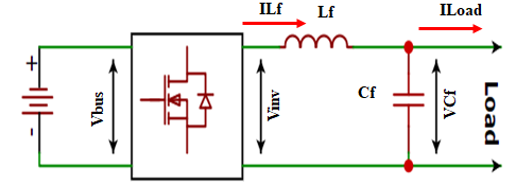##### (31)
$L_{f}\dfrac{di_{Lf}}{dt}=V_{i nv}-V_{Cf}$

##### (32)
$C_{f}\dfrac{dv_{Cf}}{dt}=I_{Lf}-I_{Load}$

The small-signal stability model of the employed VSI with LC filter can be expressed using the same procedure and technique as the average small signal stability modeling used in the PFC converter stage as (33).

##### (33)
$\left[\begin{array}{c}\dot{i}_{L f} \\ \dot{v}_{C f}\end{array}\right]=$ $\left[\begin{array}{c}0-\frac{1}{L_{f}} \\ \frac{1}{C_{f}} \frac{1}{Z_{L_{o a d}} C_{f}}\end{array}\right]\left[\begin{array}{c}i_{L f}^{*} \\ v_{C f}^{*}\end{array}\right]+\left[\begin{array}{c}\frac{1}{L_{f}} \\ 0\end{array}\right] V_{i n v}$

### 3.3 Control Circuits Design of the VSI Stage

Fig 12 depicts the control circuit of the designed VSI with LC filter, which includes the control loops for the load (filter capacitor) voltage and the filter inductor current. The performance of both loops is controlled by PI controllers designed with voltage and current transfer functions obtained from the VSI's small-signal model and the Ziegler Nichols tuning technique for closed-loop control systems.

Fig. 12. The structure of the control system for VSI Stage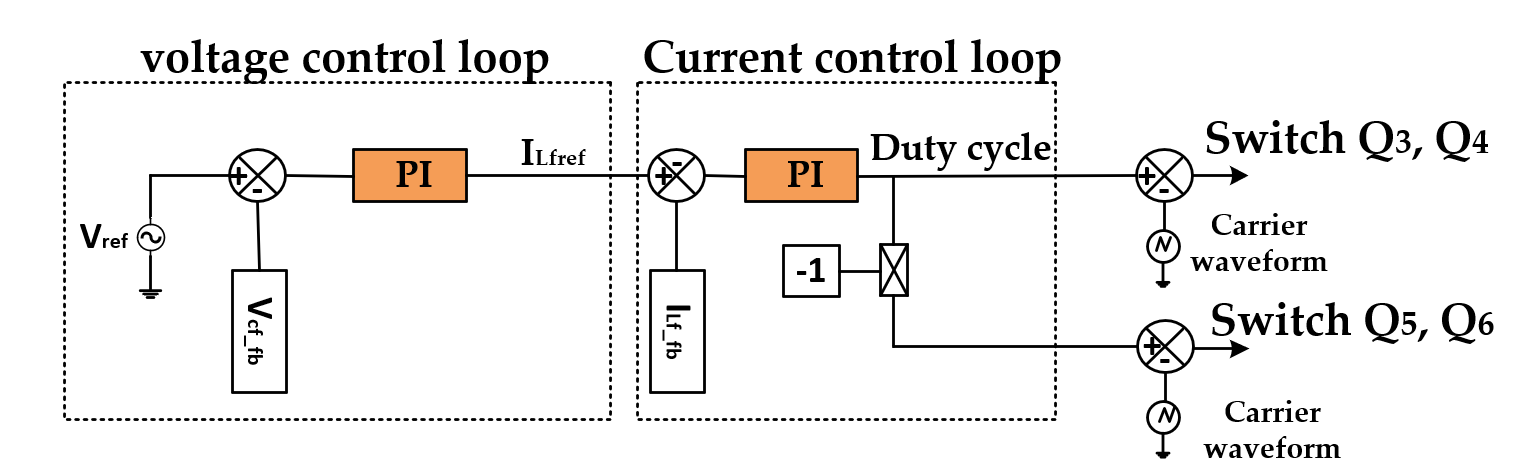Using the small signal model obtained in (33), the transfer functions (TF) of the filter capacitor voltage and filter inductor current systems for the VSI can be expressed as (34), (35).

##### (34)
$G_{v Cf}(s)=\dfrac{v_{Cf}^{*}(s)}{i_{Lf}^{*}(s)}=\dfrac{ Z_{load}}{ Z_{load} C_{f}s-1}$

##### (35)
$G_{i Lf}(s)=\dfrac{i_{Lf}^{*}(s)}{d\in v^{*}(s)}≅\dfrac{V_{bus}}{s L_{f}}$

where the load is represented as RL load with impedance $Z_{Load}$= R+sL in the s-domin.

Fig 13 shows the block diagrams of the VSI stage's closed-loop voltage and current control loops. The Ziegler Nichols tuning method for closed-loop systems with step input and sustained oscillation at the output is used to obtain the parameters of the PI controllers in both control loops.

Fig. 13. Block diagrams of the VSI closed loop control systems: (a) voltage loop; (b) current loop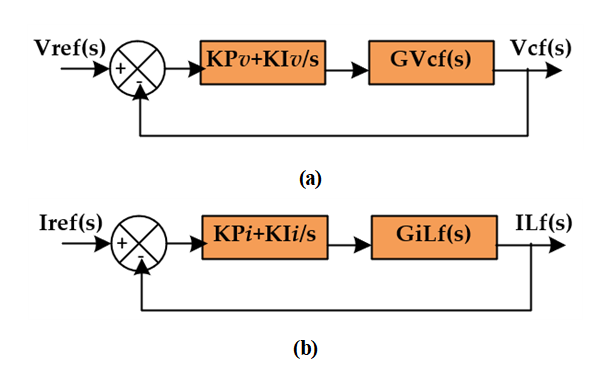Fig. 15. Closed loop Ziegler Nichols sustained oscillation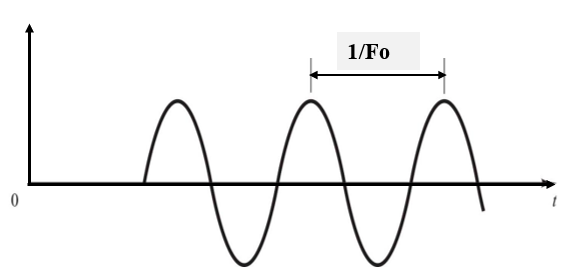Fig 14 depicts the flowchart of the Ziegler Nichols tuning technique used to calculate the PI controller parameters for both control loops. Fig 15 depicts the sustained oscillation of the Ziegler Nichols tuning method during which the controller parameters are calculated.

Table 4 shows the maximum proportional gains, frequency at sustained oscillation, and calculated $K_{P}$, $K_{I}$ parameters for the voltage and current control loops of the VSI stage using the Ziegler Nichols tuning technique.

Table 4. VSI controllers parameters values

 Voltage loop $KP_{v_Max}$ = 2.20 $F_{ov}$ = 275Hz $KP_{v}$ = 1 ;$KI_{v}$ = 334 Current loop $KP_{i_Max}$ = 1.80 $F_{oi}$ = 6667Hz $KP_{i}$ = 0.8 ;$KI_{i}$ = 8000

## 4. Simulation Results and Discussion

PSIM simulation software is used to test the performance of the proposed AC-AC power supply with the designed power components shown in Table 5 and the designed voltage and current control loops for the PFC and the VSI stages.

Table 5. Designed Power component values

 Component Value Unit Boost indictors ($L_{1}$, $L_{2}$) 240 uH Bulk capacitor ($C_{bulk}$) 100 uF Filter inductance ($L_{f}$) 3 mH Filter capacitor ($C_{f}$) 20 uF

The EMI filter of the PFC stage is implemented as a CLC filter with a common-mode inductor of 100uH and 1nF capacitors as shown in Fig 2.

The waveforms of voltages, currents, and power factor of the proposed two-stage power supply are simulated using the same inductive loading condition (R=78ohm, L=0.2H) and varying input supply voltages from minimum to maximum voltage. Fig 16 shows the performance with a rated input voltage of 220V RMS, demonstrating that the PFC stage's designed voltage control loop successfully regulated the DC bus voltage to the desired value of 390V, and the PFC stage's designed current control loops successfully maintain the phase difference between the supply voltage and current waveforms at about 8 degrees, providing supply with an input PF of 99.05% under inductive loading conditions with a PF of 72%.

Fig. 16. Voltages, currents, and PF waveforms with rated input supply voltage (220V RMS)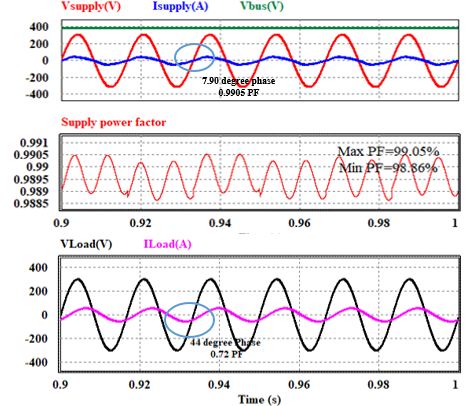Fig 17 shows the proposed power supply performance at 260V RMS maximum input supply voltage. it can be observed that, the DC bus voltage is also regulated at 390V and the load voltage is constant at 220V RMS when the input voltage is at its maximum. Also, with the same inductive loading condition and PF of 72%, increasing the supply voltage from 220V to around 260V reduces the supply PF from 99.05% to around 97.70%.

Fig. 17. Voltages, currents, and PF waveforms with maximum input supply voltage (260V RMS)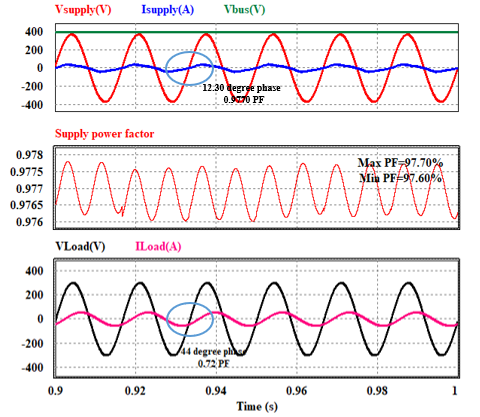Fig 18 depicts the proposed power supply performance when the input supply voltage is set to 180V RMS, demonstrating that the DC bus voltage is also regulated at 390V and the load voltage is constant at 220V RMS with a minimum input voltage. In addition, with the minimum supply voltage condition, the supply input PF increases to approximately 99.58%.

Fig. 18. Voltages, currents, and PF waveforms with minimum input supply voltage (180V RMS)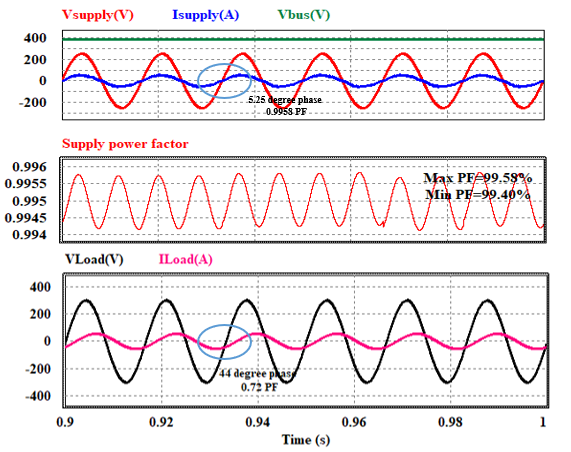With the rated supply voltage of 220V RMS and with different inductance loading conditions, the supply PF, load PF and the power efficiency of the designed two-stage power supply are measured and depicted as shown in Fig 19, which shows that with loading condition with PF of (60-80)%, the supply power factor is more than 99% and the power conversion efficiency is more than 90%.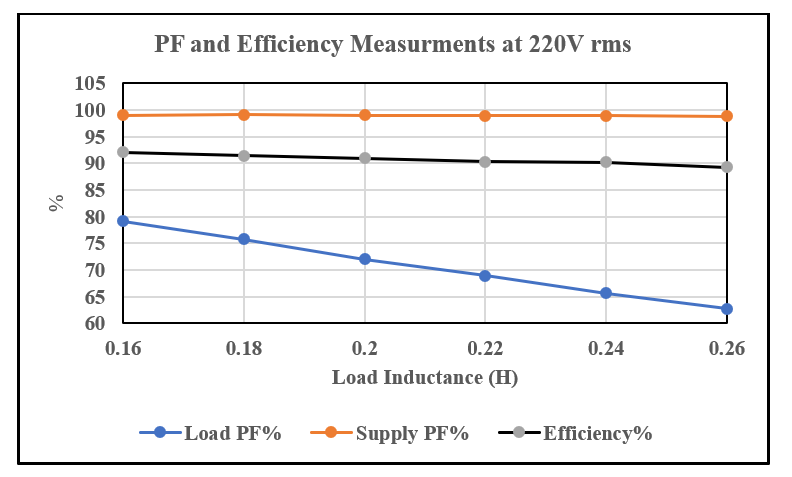The supply currents THD contents are determined and depicted in Fig. 20 under various inductance loading situations and different supply voltage conditions. With supply voltages of 180V and 220V, the designed power supply provides input supply current with a THD of less than 10% under loading conditions with PF ranging from 60% to 80%. With a 60% PF load condition, the THD content in the supply current increases to about 15% when the specified power supply operates at the maximum supply voltage limit of 260V RMS.

Fig. 20. Supply current THD measurements with different loading condition and different supply voltage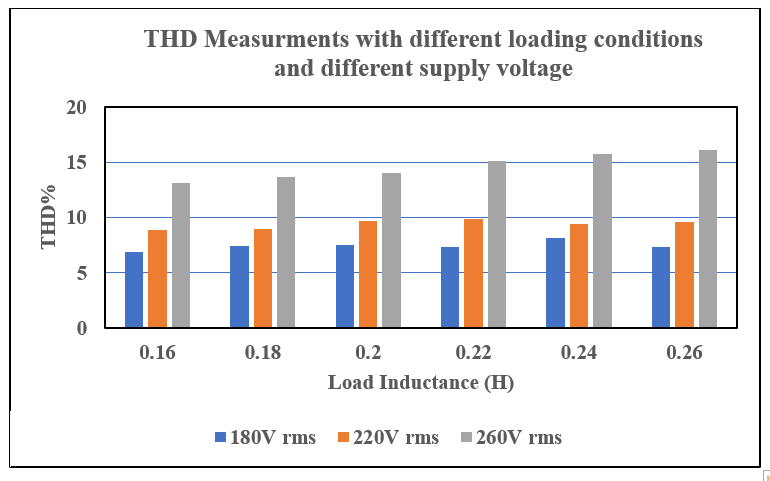## 5. Conclusion

This paper proposes a two-stage AC-AC power supply consisting of a PFC converter and a VSI with an LC filter circuit that is suitable for supplying power to inductive loads with a high input power factor, low THD, and high efficiency. The proposed power supply two-stage small-signal models are derived based on average small-signal stability modeling, and based on the derived models, the voltage and current control loops of the power supply PFC and VSI stages are optimally designed to maintain the supply PF at the highest value and regulate the DC bus voltage and load voltage at the desired values with different loading conditions and different input supply voltages, as shown in the simulations. The two-stage power supply with a wide input voltage range from 180V to about 260V RMS and 99% PF for the inductive industrial loads is achieved in this work.

### Acknowledgements

This research was funded by grant (2018R1D1A3B070 4376413) from National Research Foundation of Korea (NRF) and supported by Education and Research promotion program of KOREATECH in 2021.

### References

1
Ahmed A. AbdElhafez, H. Alruways Saud, A. Alsaif Yazeed, F. Althobaiti Mutlaq, B. AlOtaibi Abdulmohsen, A. Alotaibi Naif, May 2017, Reactive power problem and solutions: an overview, Journal of Power and Energy Engineering, Vol. 5, No. 5, pp. 40-542
Y. -W. Liu, S. -H. Rau, C. -J. Wu, W. -J. Lee, Jan 2018, Improvement of Power Quality by Using Advanced Reactive Power Compensation, IEEE Trans. on Industry Applications, Vol. 54, No. 1, pp. 18-243
Jun Qi, Zhao Wenbin, Bian Xiaoyan, Oct 2020, Comparative study of SVC and STATCOM reactive power compensation for prosumer microgrids with DFIG-based wind farm integration, IEEE Access, Vol. 8, pp. 209878-2098854
Yu-Wei Liu, Shiuan-Hau Rau, Chi-Jui Wu, Wei-Jen Lee, Aug 2017, Improvement of power quality by using advanced reactive power compensation, IEEE Trans. on Industry Applications, Vol. 54, No. 1, pp. 18-245
Ronita Pawn, Feb 2016, Comparative study of power system stability enhancement of various IEEE Bus System using SSSC, Int. J. Engineering Research & Technology, Vol. 5, No. 2, pp. 359-3636
Lei Wang, Xiaoqing Han, Chunguang Ren, Yu Yang, and Peng Wang, Mar 2017, A modified one-cycle-control-based active power filter for harmonic compensation, IEEE Trans. on Industrial Electronics, Vol. 65, No. 1, pp. 738-7487
S. Bosch, J. Staiger, H. Steinhart, June 2018, Predictive Current Control for an Active Power Filter With LCL-Filter, in IEEE Transactions on Industrial Electronics, Vol. 65, No. 6, pp. 4943-49528
Y. Kim, W. Sung, B Lee, Aug 2014, Comparative Performance Analysis of High Density and Efficiency PFC Topologies, IEEE Trans. Power Electron, Vol. 29, No. 6, pp. 2666-26799
Ahmed H. Okilly, Jeihoon Baek, Aug 2021, Optimal Design Analysis with Simulation and Experimental Performance Investigation of High-Power Density Telecom PFC Converters, Applied Sciences, Vol. 11, No. 17, pp. 791110
H. Xu, D. Chen, F. Xue, X Li, Sep 2018, Optimal design method of interleaved boost PFC for improving efficiency from switching frequency, boost inductor, and output voltage, IEEE Trans. on Power Electronics, Vol. 34, No. 7, pp. 6088-610711
G. Aiello, M. Cacciato, S. Messina, M Torrisi, Mar 2018, A high efficiency interleaved PFC front–end converter for EV battery charger, Commun.-Sci. Lett. Univ. Zilina, Vol. 20, No. 1, pp. 86-9112
Gauravkumar P. Prajapati, Archana G. Thosar, Arbind Kumar, 2021, Unity Power Factor Control using Interleaved Boost Converter for Induction Motor Drive, In 47th IECON Annual Conference of the IEEE Industrial Electronics Society, pp. 1-613
Kwang-Man Kim, Eung-Ho Kim, Jong-Woo Choi, July 2018, Control of Motor Drives Fed by PFC Circuits without DC-Link Electrolytic Capacitors, Journal of Power Electronics, Vol. 18, No. 4, pp. 1067-107414
Tao Jing, Alexander S. Maklakov, 2018, A review of voltage source converters for energy applications, In International Ural Conference on Green Energy (UralCon), pp. 275-28115
Mohammad Reza Miveh, Mohd Fadli Rahmat, Ali Asghar Ghadimi, Mohd Wazir Mustafa, July 2015, Power quality improvement in autonomous microgrids using multi-functional voltage source inverters: a comprehensive review, Journal of Power Electronics, Vol. 15, No. 4, pp. 1054-1065## 저자소개

In 2012 and 2017, he got his B.Sc. (with honors) and M.Sc. degrees in electrical engineering from Assiut University in Egypt.

He has been an assistant lecturer at the electrical engineering department of Assiut University in Egypt since 2012.

Since 2019, he has been studying for a Ph.D. at Korea University of Technology and Education in South Korea.

Power electronics, PFC, and VSI circuits, analog and digital control circuits design, power system stability, HVDC and FACTS applications are among his research interests.

In 2006, he got M.S. degrees in electrical engineering from the University of WisconsinMadison in Madison, WI, and in 2009, he received a Ph.D. in electrical engineering from Texas A&M University in College Station, TX.

He worked for Amotech in Seoul from 1998 to 2000. From 2000 to 2003, he worked as a Senior Research Engineer at Samsung Electromechanics in Suwon, Korea.

He served as a Principal Engineer at Samsung Advanced Institute of Technology in Youngin, Korea, from 2010 to 2013.

From 2014 to 2016, he worked as a Senior Research Engineer at the Korea Railroad Research Institute in Uiwang, Korea.

He has been an Assistant Professor at Korea University of Technology and Education in Cheonan, Korea, since 2017.

Design and analysis of electrical machines, variable speed drives for traction systems, and novel power conversion topology are two of his current research interests and experiences.